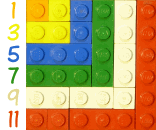# Squares and Odd Numbers

Add up odd numbers from 1 onwards and you get square numbers!

• 1 is a square number (= 1 × 1)
• 1 + 3 = 4, and 4 is a square number (= 2 × 2)
• 1 + 3 + 5 = 9, and 9 is a square number (= 3 × 3)
• etc!

Like this:

Odd
Number
Running
Total

1 1 = 1 × 1
3 4 = 2 × 2
5 9 = 3 ×3
7 16 = 4 × 4
9 25 = 5 × 5
11 36 = 6 × 6
etc...

### Is this some kind of magic?Not really, just look at this picture:

• 1 is a square (a 1×1 square)
• Add 3 and you get a 2×2 square
• Add 5 and you get a 3×3 square
• etc

It is good to know this, as it may help you solve things one day.

There are more such tricks, see Partial Sums.